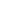Nikesh took a loan of Rs. 1500 with simple interest for as many years as the rate of interest. If he paid Rs.540 as interest at the end of the loan period, what was the rate of interest?

a) 5%
b) 6%
c) 8%
d) 9%

 6%. Solution Let rate = R% Then, Time, T = R years P = Rs.1500 SI = Rs.540==> 540 = (1500 x R x R)/100 ==> 540 = 15R2 R2 = 540/15 R = 6
Share :
+
Next
« Prev
Prev
Next »